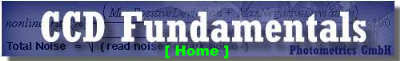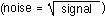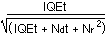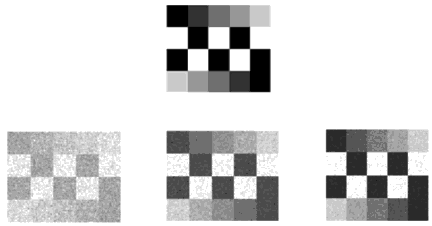##Signal-to-Noise Ratio (SNR)

Signal-to-noise ratio (SNR) describes the quality of a measurement. In CCD imaging, SNR refers to the relative magnitude of the signal compared to the uncertainty in that signal on a per-pixel basis. Specifically, it is the ratio of the measured signal to the overall measured noise (frame-to-frame) at that pixel. High SNR is particularly important in applications requiring precise light measurement.

Photons incident on the CCD convert to photoelectrons within the silicon layer. These photoelectrons comprise the signal but also carry a statistical variation fluctuations in the photon arrival rate at a given point. This phenomenon is known as Photon noise and follows Poisson statistics. Additionally, inherent CCD noise sources create electrons that are indistinguishable from the photoelectrons. When calculating overall SNR, all noise sources need to be taken into consideration:

Photon noise refers to the inherent natural variation of the incident photon flux. Photoelectrons collected by a CCD exhibit a Poisson distribution and have a square root relationship between signal and noise.Read noise refers to the uncertainty introduced during the process of quantifying the electronic signal on the CCD. The major component of readout noise arises from the on-chip preamplifier.

Dark noise arises from the statistical variation of thermally generated electrons within the silicon layers comprising the CCD. Dark current describes the rate of generation of thermal electrons at a given CCD temperature. Dark noise, which also follows a Poisson relationship, is the square root of the number of thermal electrons generated within a given exposure. Cooling the CCD from room temperature to -25°C will reduce dark current by more than 100 times. In addition, many scientific-grade CCDs employ multi-pinned-phase (MPP) technology to even further reduce dark current.

Taken together, the SNR for a CCD camera can be calculated from the following equation:where:

I = Photon flux (photons/pixel/second)
QE = Quantum efficiency
t = Integration time (seconds)
Nd = Dark Current (electrons/pixel/sec)
Nr = Read noise (electrons RMS/pixel)

Under low-light-level conditions, read noise exceeds photon noise and the image data is said to be "read-noise limited." The integration time can be increased until photon noise exceeds both read noise and dark noise. At this point the image data is then said to be "photon limited."

An alternative means of raising the SNR is to use a technique known as binning. Binning is the process of combining charge from adjacent pixels in a CCD during readout into a single "superpixel." Binning neighboring pixels on the CCD array may allow one to reach a photon-limited signal quicker at the expense of spatial resolution.

Once you have determined acceptable values for SNR, integration time, and the degree to which you are prepared to bin pixels, the above equation can be solved for the minimum photon flux required. This is, therefore, the lowest light level that can be measured for given experimental conditions and camera specifications.Visual impact of increasing SNR of a typical test pattern.